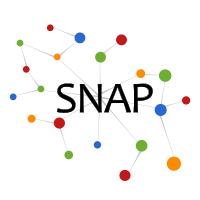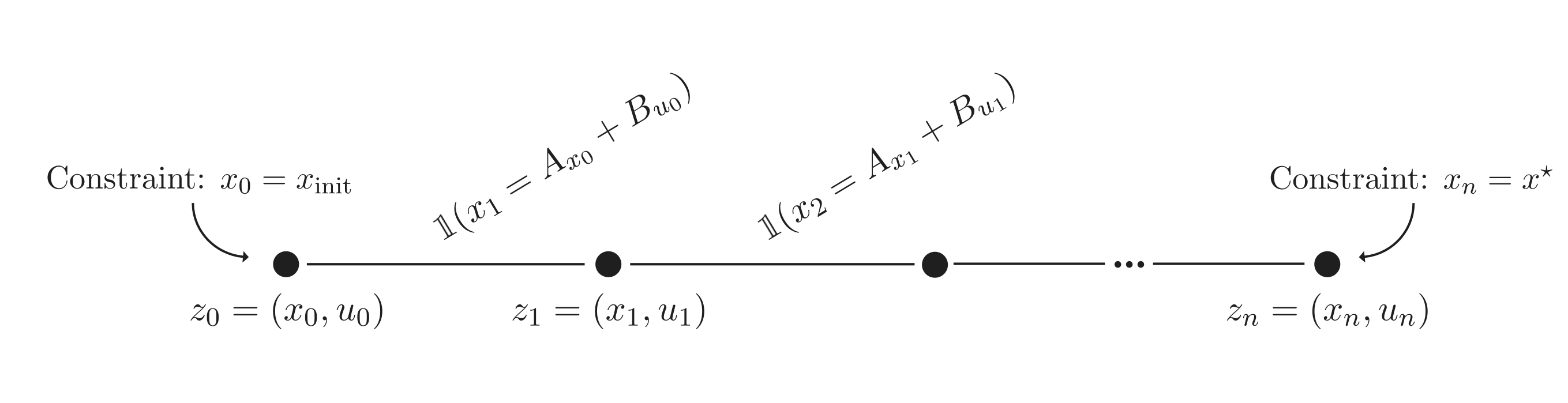# SnapVX: Network-Based Optimization Solver

SnapVX is a python-based convex optimization solver for problems defined on graphs. For problems of this form, SnapVX provides a fast and scalable solution with guaranteed global convergence. It combines the graph capabilities of Snap.py with the convex solver from CVXPY, and is released under the BSD Open-Source license.

SnapVX is a convex optimization solver for problems which are defined on a graph. Given a vertex set $\mathcal{V}$ and edge set $\mathcal{E}$, we solve the following optimization problem:

$$\mbox{minimize} \sum\limits_{i \in \mathcal{V}} f_i(x_i) + \sum\limits_{(j,k) \in \mathcal{E}} g_{jk}(x_j, x_k)$$

The variables are $x_1,x_2,\ldots,x_m$. Here, $x_i \in \mathbb{R}^p$ is the variable and $f_i$ the cost function at node $i$; $g_{jk}$ is the cost function associated with undirected edge $(j,k)$. When the cost functions are convex, SnapVX provides:

• A solution based on the alternating direction method of multipliers (ADMM) that is guaranteed to converge to the global optimum for any problem of this form.
• A fast, scalable algorithm which is capable of being distributed across multiple cores of a single machine.
• An integration of Snap.py and CVXPY.

Prerequisites:
Snap.py
CVXPY

Instructions:
See the README for instructions for how to install and test SnapVX

## Online Documentation and Resources

### Github Repository

The following development repositories are available for SnapVX

### Other Resources

• Presentation on Applying SnapVX to Real-World Problems
• CVXPY, the general convex optimization solver used to solve the ADMM subproblems, can be found here. For more information, please see the documentation on the website or contact Steven Diamond with any questions.
• To define and store the networks, SnapVX uses Snap.py. The Snap.py documentation provides detailed information on how the underlying framework works.

## Motivation

Convex optimization has become an increasingly popular way of modeling problems in many different fields. However, as datasets get larger and more intricate, classical methods of convex analysis, which often rely on interior point methods, begin to fail due to a lack of scalability. The challenge of large-scale optimization lies in developing methods general enough to work well independent of the input, while being capable of scaling to the immense datasets that today’s applications require. Therefore, it is necessary to formulate general classes of convex optimization solvers, ones that can apply to a variety of interesting and relevant problems, and to develop algorithms for reliable and efficient solutions.

By focusing on examples of the form $\mbox{minimize} \sum\limits_{i \in \mathcal{V}} f_i(x_i) + \sum\limits_{(j,k) \in \mathcal{E}} g_{jk}(x_j, x_k)$, we can solve a variety of common problems in optimization. Putting a large class of problems under a unified framework has many benefits. This is intended to be a general-use solver, taking advantage of ADMM to solve problems too large to be computed using a centralized approach. We see several examples that fit into this form in the applications section below.

We elect to build a solver that runs on a single large memory machine rather than in a distributed computing environment. This is for several reasons. First, it provides simplicity and "out-of-the-box" functionality, including being able to run on standard laptops for smaller problems. Second, large memory machines are increasingly becoming a viable and affordable alternative when performing large-scale data processing. Most real-world problems can fit comfortably in a single "big-memory" server. Even if the raw data does not fit, data cleaning and manipulation often results in a significant reduction in space that allows the relevant data to fit into memory. Finally, our ADMM-based solution is a message-passing algorithm which relies on many small messages being sent very quickly, so it is not particularly well-suited for large and distributed computing clusters (even though it is fully possible to implement the algorithm in a distributed environment).

## Syntax

We show three basic examples to demonstrate how to set up and solve a SnapVX problem. Please also see the Snap.py and CVXPY reference pages, since SnapVX relies on their syntax where necessary. The first sets up a basic problem with only 2 nodes. The second builds a random graph with random objectives, and the final example demonstrates how to bulk load data from a CSV file.

Example 1. We first look at a simple example with two nodes and one edge between them. The two nodes have different objectives, both in $\mathbb{R}^1$, and the edge objective penalizes the square of the difference of the variables.


import snapvx
import cvxpy # Note: it is not necessary to import cvxpy, as all cvxpy functionality
# is already integrated into SnapVX. It is shown here for verbosity

# Create new graph
gvx = snapvx.TGraphVX()

# Use CVXPY syntax to define a problem
x1 = cvxpy.Variable(1, name='x1')
obj = cvxpy.square(x1)
# Add Node 1 with the given objective, with the constraint that x1 <= 10

# Similarly, add Node 2 with objective |x2 + 3|
x2 = cvxpy.Variable(1, name='x2')
obj2 = cvxpy.abs(x2 + 3)

# Add an edge between the two nodes,
# Define an objective, constraints using CVXPY syntax
gvx.AddEdge(1, 2, Objective=cvxpy.square(cvxpy.norm(x1 - x2)), Constraints=[])

gvx.Solve() # Solve the problem
gvx.PrintSolution() # Print entire solution on a node-by-node basis
print "x1 = ", x1.value, "; x2 = ", x2.value # Print the solutions of individual variables



Example 2: Random Graph. Next, we look at a slightly larger example. We build a random graph (using GenRndGnm), set each node objective to the square loss from a random point in $\mathbb{R}^{10}$, and use laplacian regularization on the edges.


from snapvx import *
import numpy

# Helper function to define edge objectives
# Takes in two nodes, returns a problem defined with the variables from those nodes
def laplace_reg(src, dst, data):
return (sum_squares(src['x'] - dst['x']), [])

# Generate random graph, using SNAP syntax
numpy.random.seed(1)
num_nodes = 10
num_edges = 30
n = 10
snapGraph = GenRndGnm(PUNGraph, num_nodes, num_edges)
gvx = TGraphVX(snapGraph)

# For each node, add an objective (using random data)
for i in range(num_nodes):
x = Variable(n,name='x') # Each node has its own variable named 'x'
a = numpy.random.randn(n)
gvx.SetNodeObjective(i, square(norm(x-a)))

# Set all edge objectives at once (Laplacian Regularization)

# Solve in verbose mode (using ADMM)
gvx.Solve(Verbose=True)
gvx.PrintSolution()

gvx.PrintSolution()

# Print only the solution for 'x' at Node 1
print "Solution at Node 1 is", gvx.GetNodeValue(1,'x')



Example 3: Bulk Loading. Finally, we look at how we can bulk load the relevant data from a text file


from snapvx import *

# Helper function for node objective
# Takes in a row from the CSV file, returns an optimization problem
def node_obj(data):
x = Variable(1,name='x')
return norm(x - float(data))

# Helper function for edge objective
def laplace_reg(src, dst, data):
return sum_squares(src['x'] - dst['x'])

# Load in Edge List to build graph with default node/edge objectives

# Takes one row of the CSV, uses that as input to node_obj
# There is also an (optional) input of specifying which nodes each row of the CSV refers to

# Bulk load edge objectives for all edges

gvx.Solve()
gvx.PrintSolution()



## Potential Applications

Consensus - The consensus problem is often written as

$$\mbox{minimize} \sum\limits_{i} f_i(x)$$

This can be formulated in SnapVX as a problem on a network, where each node i has objective function $f_i(x_i)$, but we are constrained to solving for a common value of x as the solution at every node. We solve it by building any connected graph (so there is at least one path from every node to all other nodes), setting each node's local optimization variable function as $f_i(x_i)$. We then set the edge objectives to $\mathbf{1}(x_j = x_k)$. And so, the problem becomes

$$\mbox{minimize} \sum\limits_{i \in \mathcal{V}} f_i(x_i) + \sum\limits_{(j,k) \in \mathcal{E}} \mathbf{1}(x_j = x_k)$$

Regularization - Many types of regularization are natural extensions of the original SnapVX formula. For Laplacian Regularization, set the edge objective equal to $\| x_j - x_k\|_2^2$. The network lasso (see References) is very similar, except the edge objective is instead $\| x_j - x_k\|_2$.

Exchange - The exchange problem is defined as \begin{align} &\mbox{minimize} &\sum\limits_{i = 1}^N f_i(x_i) \\ &\mbox{subject to} &\sum\limits_{i =1}^N x_i = 0. \end{align} To solve this, connect all $N$ nodes (each with objective $f_i(x_i)$) to a single dummy node. This dummy node has variables $z_1, \ldots, z_N$. Each edge is a consensus constraint that $x_i = z_i$, and the dummy node's objective is the indicator function that $\sum\limits_{i =1}^N z_i = 0$.

Control Systems - A basic control system is defined by the update formula $$x^{t+1} = A x^t + B u^t,$$ where $x$ is the state, $u$ is the input (which we can choose), and $A, B$ are constant matrices. Often, we will want to reach some state $x^\star$ by step $N$. We can model this as a linear network, where each of the $N$ steps is its own node (node $i$ is connected to $i+1$ and $i-1$). The optimization parameter at each node is $z_i = (x_i, u_i)$. Each edge represents a constraint that $x^{t+1} = A x^t + B u^t$. The first node has a constraint that $x_0 = x_{\mathrm{init}}$, the initial state, and the last node, node $N$, is constrained by $x_N = x^\star$. The overall problem is described in the picture below. From here, it is easy to add other objectives and constraints to represent other goals, such as minimizing total "fuel usage" ($\|u\|$) or not deviating too far from prescribed bounds on the path.Fixed Routing - Consider a set of paths on a graph (for example, traffic routes in a communication network). Each route wants to maximize its throughput while traversing through a series of nodes and edges, but there are constraints on the total allowable flow at each node (i.e. the router can only process a certain number of packets per second). This is often written as \begin{align} &\mbox{maximize} &\sum\limits_{i = 1}^N u_i(f_i) \\ &\mbox{subject to} &R f \leq c, \end{align} where the $u$'s are the utility functions, $R$ is the routing matrix (a boolean matrix denoting which flows go through which nodes), and $c$ is the constraint vector. Rather than building the entire flow network, we use a bipartite graph instead. One side of this graph contains each of the original nodes, and the other side contains each of the routes. There is an edge between node $i$ and route $j$ if $j$ goes through $i$ (or $R_{ij} = 1$). The edges represent consensus constraints between the two sides of the bipartite graph. The node objective at the node representing route $i$ is to maximize $u_i(f_i)$. The other side of the bipartite graph, representing the original nodes in the routing network, just have constraints that the sum of flows through it is less than $c_i$.PageRank - The PageRank problem can be defined as a convex optimization problem split up across a graph (for details: http://jmlr.org/proceedings/papers/v32/gleich14.pdf). A working implementation is included in the Examples folder of the SnapVX download.

...and many more!

## Contributors

The following people contributed to SnapVX (in alphabetical order):

Stephen Boyd
Steven Diamond
David Hallac
Jure Leskovec
Youngsuk Park
Abhijit Sharang
Rok Sosic
Christopher Wong

### Getting Involved

You are encouraged to try SnapVX for your data science and convex optimization needs. If you wish to contribute to the project, see here for a list of open problems and potential new features. If you find any bugs, please report them here. Additionally, to give us any other feedback, feel free to email David Hallac to get in touch!

## References

SnapVX: A Network-Based Convex Optimziation Solver. D. Hallac, C. Wong, S. Diamond, A. Sharang, R. Sosic, S. Boyd, J. Leskovec. Journal of Machine Learning Research (JMLR) , 2017.

Related Work
Network Lasso: Clustering and Optimization in Large Graphs. D. Hallac, J. Leskovec, S. Boyd. ACM SIGKDD International Conference on Knowledge Discovery and Data Mining (KDD) , 2015.
Code from Network Lasso paper available on Github.